Courses

Test: Shapes - 1

10 Questions MCQ Test Mathematics for Class 1 | Test: Shapes - 1

Description
Attempt Test: Shapes - 1 | 10 questions in 15 minutes | Mock test for Class 1 preparation | Free important questions MCQ to study Mathematics for Class 1 for Class 1 Exam | Download free PDF with solutions
QUESTION: 1

Choose the correct option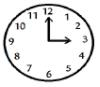Solution: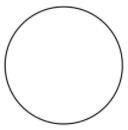A circle is a round shaped figure that has no corners or edges.
In geometry, a circle can be defined as a closed, two - dimensional curved shape.

So, This figure is circle.

QUESTION: 2

Circle has __________ corners

Solution:

A corner is where 2 sides meet. E.g. a triangle has 3 straight sides and 3 corners, whereas a circle has 1 curved side but no corners.

QUESTION: 3

Which shape has 3 sides and 3 corners?

Solution: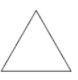A triangle is a polygon with three edges and three vertices. It is one of the basic shapes in geometry.

QUESTION: 4

The object that can roll

Solution:

The object which has a curved surface that is all circular objects can roll. Examples: Ball, lemon, orange, apple, football, etc.

QUESTION: 5

Identify the shape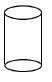Solution:

The cylinder can be defined as a solid geometrical figure with straight parallel sides and a circular or oval cross-section. Examples are: Pipes. Cold drink cans.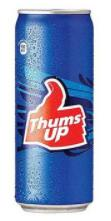QUESTION: 6

Complete the pattern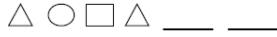Solution:

The definition of a pattern is someone or something used as a model to make a copy, a design, or an expected action. An example of a pattern is the paper sections a seamstress uses to make a dress; a dress pattern. An example of a pattern is polka dots. An example of a pattern is rush hour traffic; a traffic pattern.

So, Option c is the correct answer.

QUESTION: 7

Ludo is an example of

Solution:

A plane figure with four equal straight sides and four right angles.

So, the Ludo is an example of Square.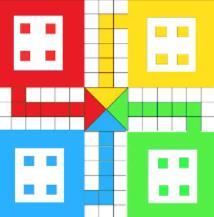QUESTION: 8

Tick the correct next pattern 10, 20, 30 A B C A B C ______ _______ _____

Solution:

Pattern is a repeated decorative design.

So, The ABC is will come in the blank.

QUESTION: 9

Identify the shape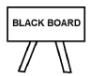Solution:

Rectangle: A rectangle is a 2D shape in , having 4 sides and 4 corners. Its two  meet at . Thus, a rectangle has 4 , each measuring 90 ̊. The opposite sides of a rectangle have the same lengths and are parallel.  Two sides are said to be parallel, when the distance between them remains the same at all points.

QUESTION: 10

Identify the shape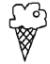Solution:

A cone is a distinctive three-dimensional geometric figure that has a flat surface and a curved surface, pointed towards the top. The pointed end of the cone is called the apex, whereas the flat surface is called the base. This is what a cone looks like: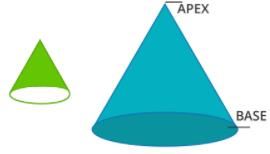Use Code STAYHOME200 and get INR 200 additional OFF Use Coupon Code

Track your progress, build streaks, highlight & save important lessons and more!

Similar Content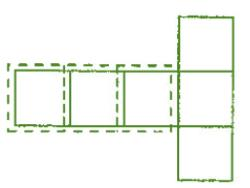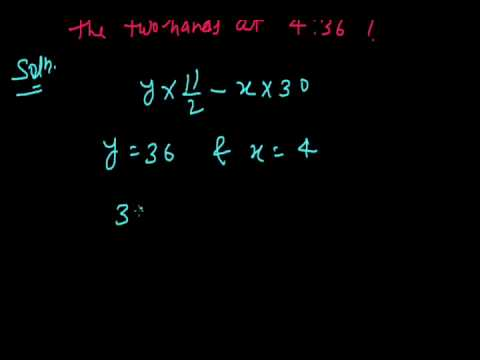Related tests